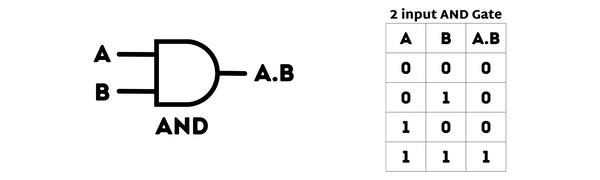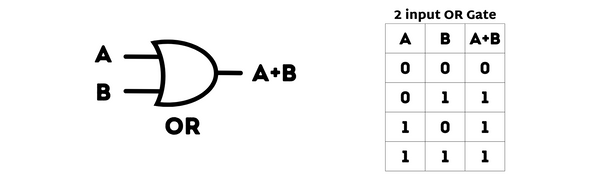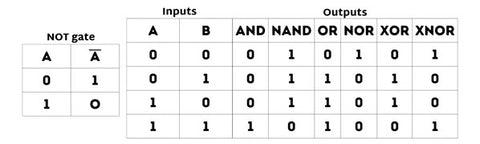# Understanding AND OR NOT Gates with simple Graphics and Truth TablesThere are seven basic logic gates: NOT, AND, OR, XOR, NOR, XNOR, and NAND.

They are essential in building more complex logic circuits, but understanding their functionality can be challenging for beginners.

In this article, we'll break down the most commonly used—AND OR NOT Gates —by including visuals, truth tables, common uses, and more.

## AND OR NOT Gates

### AND gate

An AND gate takes two input signals and produces a single output signal.It works by only producing an output signal when both input signals are present, or "high" (1).

In other words, the AND gate performs a logical "and" operation on its inputs. If either of the input signals is absent or "low" (0), then there will be no output signal.

The AND gate is often used in combination with other logic gates to create more complex circuits and perform more intricate operations.

AND gate tips
• A dot (.) is used to show the AND operation i.e. A.B. Bear in mind that this dot is sometimes omitted i.e. AB
• The AND gate has two inputs and one output. The output is 1 if both inputs are 1, and for all other cases the output is 0

### OR gate

An OR gate takes two input signals and produces a single output signal.However, the OR gate differs from the AND gate in that it only requires one of its input signals to be present or high to produce an output signal.

In other words, the OR gate performs a logical "or" operation on its inputs. Only when both input signals are absent or low will there be no output signal.

Like the AND gate, the OR gate can also be combined with other logic gates to create more complex circuits and perform more intricate operations.

OR gate tips
• A plus (+) is used to show the OR operation
• The OR gate has two inputs and one output. If at least one of the inputs is 1, then the output will be 1. If neither input is 1, the output will be 0

### NOT gate

Unlike the AND and OR gates that take two input signals, the NOT gate only takes one input signal and produces a single output signal.The NOT gate simply inverts or reverses the input signal. If the input is “high,” then the output will be “low,” and vice versa.

The NOT gate plays a crucial role in digital logic design as it can be used to negate or flip certain signals, leading to more complex operations and circuits.

#### NOT gate tips

• If the input variable is A, the inverted output is known as NOT A.  This is also shown in the outputs as A with a bar over the top.

## Basic Logic Gate symbols

Here are the symbols for the 7 basic logic gates.

We did a full write-up on how to use a Gate in Computer Science, Computer Engineering, or any of the hardware related tech fields.## AND OR NOT gates represented using the Truth Table

Tables listing all logical possibilities like this are known as truth tables. You can refer to this table to familiarize yourself with how each gate functions.While we're here, here are some basic tips on Logic gates.

Electronic logic gates are often used to implement Boolean expressions. Here’s what you need to know about this process:

• You will need a power supply in order to activate these electronic gates.
• The INPUT of a gate will have two voltages containing nominal values. These will represent the logic binary—for example, 0V correlates to 0 (low output) and 5V to 1 (high output).
• The gate OUTPUT will also contain these voltages. Typically, each gate only results in one output, but there are a few exceptions.
• It will take time for the output to respond to the input—always expect a delay in this process.

## AND, OR, NOT gates so easy a baby could understand

If you need more help understanding computer logic gates, we created a book so simple that even a baby could understand them.

It’s called Computer Engineering for Babies, check it out!